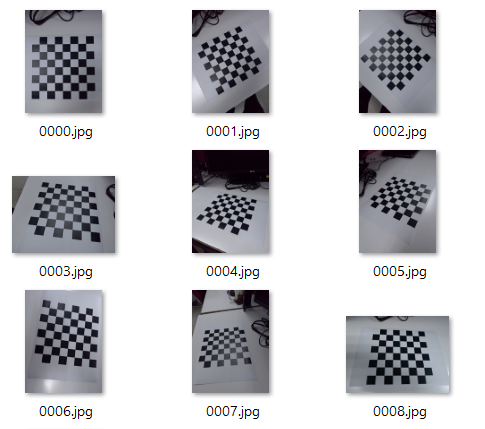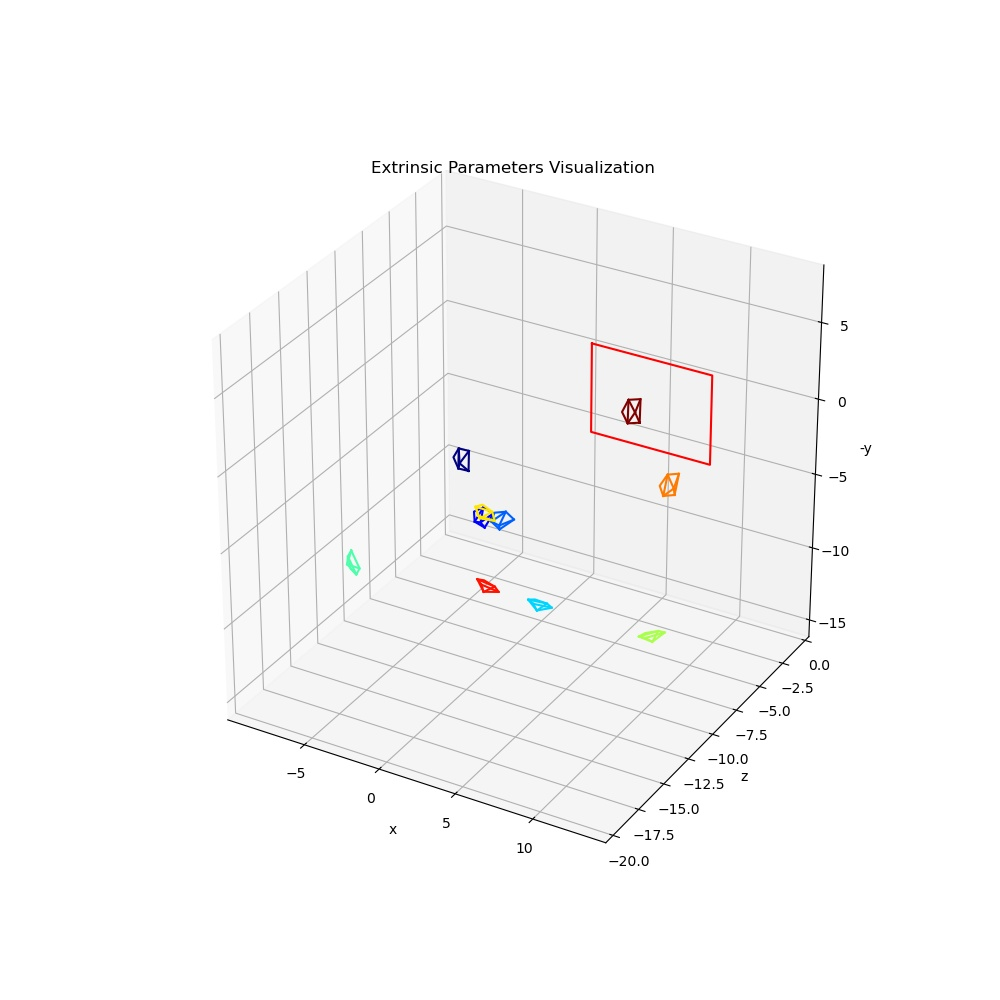## ¶ 1. 簡介

$$\mathbf{\tilde m} = \mathbf A [\mathbf R \quad \mathbf t] \mathbf {\tilde M}$$

## ¶ 2. 求 Homography

\begin{align} { \begin{bmatrix} u \\ v \\ 1 \end{bmatrix} = \mathbf A [\mathbf r1 \quad \mathbf r2 \quad \mathbf r3 \quad \mathbf t] \begin{bmatrix} X \\ Y \\ 0 \\ 1 \end{bmatrix} } \\ { = \mathbf A [\mathbf r1 \quad \mathbf r2 \quad \mathbf t] \begin{bmatrix} X \\ Y \\ 1 \end{bmatrix} } \end{align}$$\mathbf{\tilde m} = \mathbf H \mathbf {\tilde M}$$

$$\begin{bmatrix} {\mathbf {\tilde M}}^T & \mathbf 0^T & -u {\mathbf {\tilde M}}^T\\ \mathbf 0^T & {\mathbf{\tilde M}}^T &-v{\mathbf {\tilde M}}^T \end{bmatrix} \mathbf x = \mathbf L \mathbf x = 0$$

$$\begin{bmatrix} &X_1 &Y_1 &1 &0 &0 &0 &-uX_1 &-uY_1 &-u \\ &0 &0 &0 &X_1 &Y_1 &1 &-vX_1 &-vY_1 &-v \end{bmatrix}$$

$\mathbf x$ 的解就會是 the eigenvector of $\mathbf L^T \mathbf L$ associated with the smallest eigenvalue。用程式碼可能會比較直覺：

L # 2n x 9 的 numpy 矩陣
w, v, vh = np.linalg.svd(L)
x = vh[-1]


$$\mathbf H = \rho x = \rho \begin{bmatrix} x_1 &x_2 &x_3 \\ x_4 &x_4 &x_5 \\ x_6 &x_7 &x_8 \end{bmatrix}$$

$$\mathbf{\tilde m} = \rho \mathbf x \mathbf {\tilde M}$$

## ¶ 3. Intrisic Parameters 的限制

$$[\mathbf h_1 \quad \mathbf h_2 \quad \mathbf h3] = \lambda \mathbf A [\mathbf r_1 \quad \mathbf r_2 \quad \mathbf t]$$

$\lambda$ 是一個任意係數，根據 $r_1$ 和 $r_2$ 是正交 (orthonormal)，可以有以下式子：

\begin{align} {\mathbf h_1}^T {\mathbf A}^{-T} \mathbf A^{-1} \mathbf h_2 &= 0 \\ {\mathbf h_1}^T {\mathbf A}^{-T} \mathbf A^{-1} \mathbf h_1 &= \mathbf {h_2}^T \mathbf A^{-T} \mathbf A^{-1} \mathbf h_2 \end{align}

## ¶ 4. 求 Intrisic Parameters

$$\mathbf B = {\mathbf A}^{-T} {\mathbf A}^{-1} = \begin{bmatrix} B_{11} &B_{12} &B_{13} \\ B_{12} &B_{22} &B_{23} \\ B_{13} &B_{23} &B_{33} \end{bmatrix}$$

$\mathbf B$ 是一對稱矩陣，然後令 $\mathbf b = [B_{11}, B_{12} ,B_{22}, B_{23}, B_{33}, B_{11}]^T$

$\mathbf H$ 已經知道，令 $\mathbf h_i$ 指 $\mathbf H$ 的第 i 個直欄，所以 $\mathbf h_i = [h_{i1}, h_{i2} ,h_{i3}]^T$

$$\mathbf v_{ij} = [h_{i1} h_{j1}, h_{i1} h_{j2} + h_{i2} h_{j1}, h_{i2} h_{j2}, h_{i3} h_{j1} + h_{i1} h_{j3}, h_{i3} h_{j2} + h_{i2} h_{j3}, h_{i3} h_{j3}]^T$$

$$\begin{bmatrix} \mathbf v_{12}^T \\ {( \mathbf v_{11} - \mathbf v_{22})}^T \end{bmatrix} \mathbf b = \mathbf V \mathbf b = 0$$

w, v, vh = np.linalg.svd(V)
b = vh[-1]


\begin{align} v_0 &= (B_{12} B_{13} − B_{11} B_{23})/(B_{11} B_{22} − B_{12}^2)\\ λ &= B_{33} − [B_{13}^2 + v_0(B_{12}B_{13} − B_{11}B_{23})]/B_{11}\\ α &=\sqrt{λ/B_{11}}\\ β &=\sqrt{λB_{11}/(B_{11}B_{22} − B^2_{12})}\\ γ &= −B_{12} α^2 β/λ\\ u_0 &= γv_0/β − B_{13}α^2/λ\\ \end{align}

$$\mathbf A = \begin{bmatrix} α &γ &u_0 \\ 0 &β &v_0 \\ 0 &0 &1 \end{bmatrix}$$

## ¶ 5. 求 extrinsic parameters

$$\mathbf H = [\mathbf h_1 \quad \mathbf h_2 \quad \mathbf h_3] = \mathbf A [\mathbf r_1 \quad \mathbf r_2 \quad \mathbf r_3 \quad \mathbf t]$$

\begin{align} \mathbf r_1 &= \lambda \mathbf A^{-1} \mathbf h_1 \\ \mathbf r_2 &= \lambda \mathbf A^{-1} \mathbf h_2 \\ \mathbf r_3 &= \mathbf r_1 \times \mathbf r_2 \\ \mathbf t &= \lambda \mathbf A^{-1} \mathbf h_3 \\ \end{align}

## ¶ OpenCV 的 calibrateCamera 函數

ret, mtx, dist, rvecs, tvecs = cv2.calibrateCamera(objpoints, imgpoints, img_size,None,None)


from scipy.spatial.transform import Rotation as R

# A, h1, h2, h3 為已求得 numpy 矩陣

lambda_ = 1/np.linalg.norm(np.dot(np.linalg.inv(A), h1))

r1 = lambda_*np.dot(np.linalg.inv(A), h1)
r2 = lambda_*np.dot(np.linalg.inv(A), h2)
r3 = np.cross(r1, r2
r_matrix = np.array([r1, r2, r3)]).T

t = lambda_*np.dot(np.linalg.inv(A), h3)
r = R.from_matrix(r_matrix).as_rotvec()

rvecs.append(r)
tvecs.append(t)


## ¶ Extrinsic 視覺化